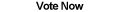Home Articles Components Code Snippets Links# True C# Random Number Generator

## .NET Random Generator Class

C#.NET comes with a built-in random number generator that is accessed through the Random class. The thing about the C# Random class however is that it generates pseudo-random numbers. That means that random numbers come from mathematical formulas that generate sequences of numbers that appear to be random...

For many C# applications a pseudo-random number generator is more than enough. The advantage being that numbers are generated quickly.

However for C# random applications that deal with things like lottery or very secure encryption, generating truly random numbers is important. That is where the true number generator comes in.

## True Random Numbers

Truly random numbers are created by observing physical phenomenas and feeding the data into a computer. The numbers generated are completely random.

Luckily for us there are free website servers that generate these kinds of true random numbers for us. One is random.org, which uses atmospheric noise to generate random numbers. The only downside to the website is that it has a quota of bytes per ip address. Luckily it is not bad at all, you get something like a million bytes and the quote is reset every once in a while...

## Connecting C# to Random.org

```http://www.random.org/integers/?num=[number of trials]&min=[min value]&max=[max value]&col=1
&base=10&format=html&rnd=new```

So you need to supply at most three parameters: the minimum value, the maximum value (inclusive as opposed to the .Net Random class), and the amount of trials.

## Using the Code

The final C# random generator source code is really simple to use. Unlike the .NET Random class, our RandomNumberGenerator class does not need to be initiated and is still perfectly thread-safe.

For example, if we want a random number between 1 and 100 the code would look like this:

`RandomNumberGenerator.GetRandomInt(1, 100)`

Back to C# Article List